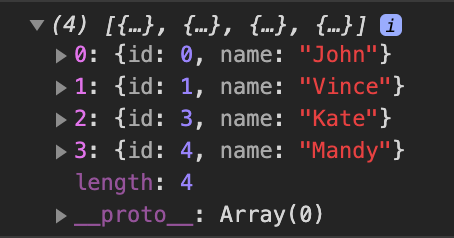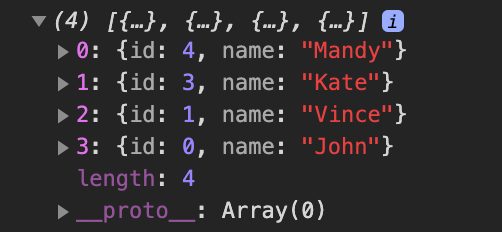# Sorting arrays with JavaScript: a deep look into the sort() method

Arrays have a lot of helpful higher-order functions that make working with them easier.

Our focus in this article will be the `sort()` method, this method has both easy and complex use cases which we'll be looking at in this article.

## First look at the `sort()` method

The assuming we have an array of fruits and we wanted to sort them alphabetically, we could do that by following this example below:

```const fruits = ['Apple', 'Pineapple', 'Orange']
fruits.sort();
console.log(fruits);
// => ["Apple", "Orange", "Pineapple"]```

Tip: if you're unfamiliar with arrow functions, read this short article

The code sample above is the easiest use-case for the `.sort()` function.

The `sort()` method like every other higher-order function accepts a function as an argument, the accepted function is called the "compare function", this compare function determines the sort order (more details later).

Before we go deep into how to use the `sort()` method, let's look at another example.

Say we have an array of numbers, and we wanted to sort them, examine the code below using the exact technique used previously.

```const digits = [1, 2, 400, 500, 3, 8];
digits.sort();
console.log(digits);
// => [1, 2, 3, 400, 500, 8]```

Not what you were expecting right? "how is 400 before 8???".

This is where the compare function comes in handy.

## The compare function

The compare function is completely optional when using the `sort()` method.

If the compare function is not supplied, the array elements are converted to strings and compared by their UTF-16 codes. That's why 400 was before 8 in the result because the 4 in 400 comes before 8 as a string.

### Using the compare function

The compare function takes two parameters, both parameters are the first two elements in the current iteration.

Now that we know why the compare function is important, let's take a look at an example where we use it.

Looking at the previous code sample again:

```const digits = [1, 2, 400, 500, 3, 8];
const compareFunc = function(a,b){
return a - b;
}

digits.sort(compareFunc);

console.log(digits);

//=> [1, 2, 3, 8, 400, 500]```

Better right?

So what exactly is happening? How does the compare function know which element to place first? Let's look into that now.

### How does the compare function work?

The two elements are compared by subtracting one from the other. When doing this, there are three outcomes.

• If comparing `a` and `b` returns a value less than 0 (negative value), `a` will be placed at a lower index, meaning that it will come before `b` in the order.
• If comparing `a` and `b` returns 0 exactly, no change in order is done between the two, but they will be placed with respect to the order elements in the array.
• If comparing `a` and `b` returns a value greater than 0 (positive value), `b` will be placed at a lower index, meaning that `b` will come before `a`.

This way we can achieve either ascending order or descending order.

From the code sample above, we've already been able to achieve an ascending order, to obtain a descending order, we can switch the operands so the values would now be positive:

```const digits = [1, 2, 400, 500, 3, 8];
const compareFunc = function(a,b){
return b - a;
}

digits.sort(compareFunc);

console.log(digits);

//=> [500, 400, 8, 3, 2, 1]```

## Complex cases in sorting

Now we know how to sort plain numerical values in an array, but what if we wanted to sort objects in an array based on a property these objects have in common.

### How to sort an array of objects based on a property's value

If we had an array of objects that looked like this:

```const users = [
{
id: 0,
name: 'John'
},
{
id: 3,
name: 'Kate'
},
{
id: 1,
name: 'Vince'
},
{
id: 4,
name: 'Mandy'
}
]```

If we wanted to sort them by their ids in ascending order, we'd do it like this:

```users.sort((a,b) => a.id - b.id)
console.log(users)```

The result would look like this:And If we wanted descending order:

```users.sort((a,b) => b.id - a.id)
console.log(users)```

The result would look like this:## Caveats

When working with the `.sort()` you have to be careful because the method doesn't create a copy of the original array, it mutates it directly, If that's not your intention, you should probably create a copy yourself first.